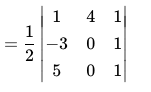The tangent and the normal drawn to the curve y = x2 - x + 4 at P(1, 4) cut the x-axis at A and B, respectively. If the length on the subtangent drawn to the curve at P is equal to the length of the subnormal, then the area of the ∆PAB(in sq units) is

1) 4

2) 32

3) 8

4) 16

Solution:

y = x2 – x + 4

Slope of the tangent P (1, 4) is

dy / dx = 2x – 1

(dy / dx)1,4 = 2 – 1 = 1

The equation of the tangent is y – 4 = 1 (x – 1)

y – x = 3 — (i)

The equation of normal at point P (1,4) is y – 4 = – 1 (x – 1)

x + y = 5 — (ii)

The tangent cuts the x-axis at A.

The coordinates of A are (- 3, 0) and the normal cuts x-axis at B and coordinates of B are (5, 0).

Area of ∆PAB= (1 / 2) |[- 4 (- 3 – 5)]|

= (1 / 2) (32)

= 16 square units(0)(0)